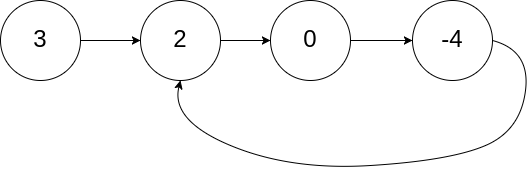## 关于数组和链表的几个必知必会的代码实现

### 数组

• 实现一个支持动态扩容的数组

• 实现一个大小固定的有序数组，支持动态增删改操作

• 实现两个有序数组合并为一个有序数组

### 链表

• 实现单链表、循环链表、双向链表，支持增删操作

• 实现单链表反转

• 实现两个有序的链表合并为一个有序链表

• 实现求链表的中间结点

## 数组题目

### Three Sum（求三数之和）

[
[-1, 0, 1],
[-1, -1, 2]
]

public List<List<Integer>> threeSum(int[] nums) {
if (nums.length < 3) {
return new ArrayList<>();
}
//排序
Arrays.sort(nums);
List<List<Integer>> result = new ArrayList<>();
for (int i = 0; i < nums.length; i++) {
if (nums[i] > 0) {
break; // 如果当前数字大于0，则三数之和一定大于0，所以结束循环（没有0或负数怎么加也不会等于0）
}
if (i > 0 && nums[i] == nums[i - 1]) {
continue; // 去重
}
int left = i + 1;
int right = nums.length - 1;
while (left < right) {
int sum = nums[i] + nums[left] + nums[right];
if(sum == 0){
while (left < right && nums[left] == nums[left+1]) {
left++; // 去重
}
while (left < right && nums[right] == nums[right-1]) {
right--; // 去重
}
left++;
right--;
} else if (sum < 0) {
left++;
} else {
right --;
}
}
}
return result;
}

### Majority Element（求众数）

public int majorityElement(int[] nums) {
if (nums.length < 1) {
return 0;
}
Arrays.sort(nums);
return nums[nums.length/2];
}

[7, 7, 5, 7, 5, 1 | 5, 7 | 5, 5, 7, 7 | 7, 7, 7, 7]

[7, 7, 5, 7, 5, 1 | 5, 7 | 5, 5, 7, 7 | 5, 5, 5, 5]

public int majorityElement(int[] nums) {
int count = 0;
Integer candidate = null;

for (int num : nums) {
if (count == 0) {
candidate = num;
}
count += (num == candidate) ? 1 : -1;
}

return candidate;
}

### Missing Positive（求缺失的第一个正数）

public int firstMissingPositive(int[] nums) {
int n = nums.length;

// 基本情况
int contains = 0;
for (int i = 0; i < n; i++)
if (nums[i] == 1) {
contains++;
break;
}

if (contains == 0)
return 1;

// nums = 
if (n == 1)
return 2;

// 用 1 替换负数，0，
// 和大于 n 的数
// 在转换以后，nums 只会包含
// 正数
for (int i = 0; i < n; i++)
if ((nums[i] <= 0) || (nums[i] > n))
nums[i] = 1;

// 使用索引和数字符号作为检查器
// 例如，如果 nums 是负数表示在数组中出现了数字 1
// 如果 nums 是正数 表示数字 2 没有出现
for (int i = 0; i < n; i++) {
int a = Math.abs(nums[i]);
// 如果发现了一个数字 a - 改变第 a 个元素的符号
// 注意重复元素只需操作一次
if (a == n)
nums = - Math.abs(nums);
else
nums[a] = - Math.abs(nums[a]);
}

// 现在第一个正数的下标
// 就是第一个缺失的数
for (int i = 1; i < n; i++) {
if (nums[i] > 0)
return i;
}

if (nums > 0)
return n;

return n + 1;
}

## 链表题目public boolean hasCycle(ListNode head) {
return false;
}
while (slow != fast) {
if (fast == null || fast.next == null) {
return false;
}
slow = slow.next;
fast = fast.next.next;
}
return true;
}

### Merge k Sorted Lists（合并 k 个排序链表）

[
1->4->5,
1->3->4,
2->6
]

public ListNode mergeKLists(ListNode[] lists) {
PriorityQueue<ListNode> queue = new PriorityQueue<>(Comparator.comparingInt(node -> node.val));
ListNode dummy = new ListNode(0);
ListNode p = dummy;
while (!queue.isEmpty()) {
ListNode node = queue.poll();
p.next = node;
p = p.next;
if (node.next != null)
}